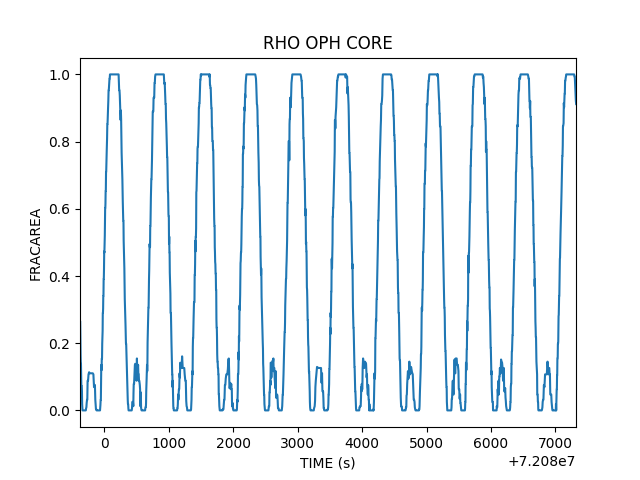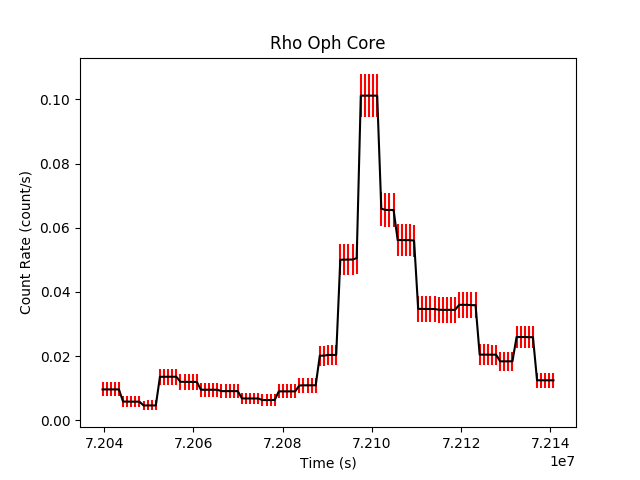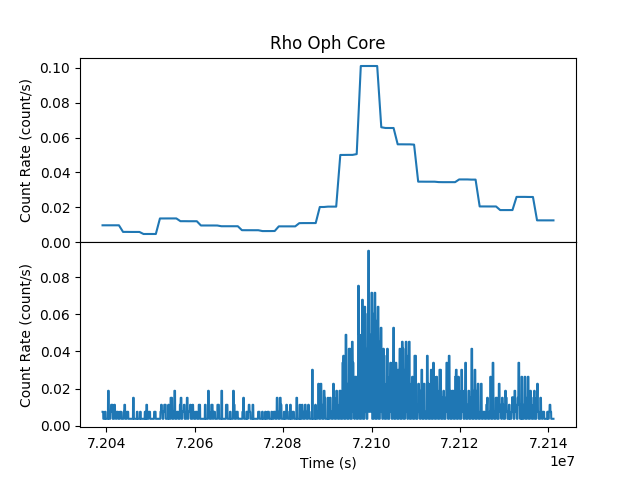# Search for Variability in a Source## Overview

#### Synopsis:

The glvary tool searches for variability using the Gregory-Loredo algorithm. It splits the events into multiple time bins and looks for significant deviations between them. The tool assigns a variability indexed based on the total odds ratio, the corresponding probability of a variable signal, and the fractions of the lightcurve which are within 3σ and 5σ of the average count rate.

For an in-depth discussion of the variability algorithm, refer to the Gregory-Loredo Variability Probability why topic in the Chandra Source Catalog website.

#### Purpose:

To determine whether a source is variable.

Last Update: 8 Apr 2019 - Updated to use matplotlib for plotting.

## Get Started

`unix% download_chandra_obsid 635 evt2,asol,msk,bpix`

## Select a Source

We need to define a source region to use in the analysis. To manually create the source region, first display the image:

```unix% ds9 acisf00635N004_evt2.fits &
```

In this example, we chose a source on ACIS-I3 (ccd_id=3), as shown in Figure 1.

### Figure 1: Source region on the event file### Figure 1: Source region on the event file

To save the regions, follow these steps:

1. Region → Save Regions... → src.reg
2. After choosing "OK" in the region filename dialog, a format dialog is opened. Set the format to "CIAO" and the coordinate system to "Physical".

The resulting region file looks like:

```unix% more src.reg
# Region file format: CIAO version 1.0
circle(4153.375,3569.25,10)
```

## Compute the Fractional Area

A normalized effective area file is needed for input to glvary. This file is created by the dither_region tool. dither_region takes a region on the sky and computes its location on the detector. When part of the region falls onto a bad-pixel, goes off the detector, or goes outside the bounds of the mask (optional) the area is decremented. Why this file is necessary is explained in further detail in the "Comparison: running glvary without dither_region" section.

dither_region also takes bad pixels into account. Users need to be sure that the ardlib parameter file is set before running dither_region

```unix% punlearn ardlib
unix% acis_set_ardlib acisf00635_000N004_bpix1.fits abs-
Updated ardlib parameter file: /home/user/cxcds_param4/ardlib.par
```

The tool requires the aspect solution for the observation and the source region as inputs. Note that if there are multiple asol1.fits file for your observation, all of them must be provided, either as a list or as a stack. The mask file is necessary for the tool to know which CCDs were used in the observation.

```unix% punlearn dither_region
unix% pset dither_region region="region(src.reg)"
unix% pset dither_region outfile=fracarea.fits

unix% dither_region
Input aspect solution or histogram file(s) (pcadf072039163N004_asol1.fits):
Region specification (region(src.reg)):
Output file name (fracarea.fits):
```

The output file, fracarea.fits, contains a fractional area for each row in the input file. The STATUS column indicates why the fraction for that row is less than 1; see the help file for an explanation of the status bits.

```unix% dmlist fracarea.fits cols

--------------------------------------------------------------------------------
Columns for Table Block AREAFRACTION
--------------------------------------------------------------------------------

ColNo  Name                 Unit        Type             Range
1   TIME                 s            Real8          72039163.6412879974: 72141147.5575280041 Time
2   EQPOS(RA,DEC)        deg          Real8          -360.0:      360.0   Sky Position
3   ROLL                 deg          Real8          -Inf:+Inf            Roll angle
4   FRACAREA                          Real8          -Inf:+Inf            Fraction area
5   AREA_CHIP_FRAC                Real8(10)      -Inf:+Inf            Region Area Fraction per chip
6   DELTA_T              s            Real8          -Inf:+Inf            Time
7   CHIP_FRAC_TIME                Real8(10)      -Inf:+Inf            Fraction of ontime per chip
8   STATUS                            Bit                              Why fraction < 1
```

The contents of the parameter file may be checked with plist dither_region.

### Figure 2: Fraction of Region Area vs. Time### Figure 2: Fraction of Region Area vs. Time

A plot showing the fraction of the region area versus time. The range of the plot has been expanded to cover a short time range so the periodicity is easy to see.

```unix% python

>>> import matplotlib.pylab as plt
>>>
>>> xx = tab.get_column("time").values
>>> yy = tab.get_column("fracarea").values
>>>
>>> plt.plot(xx,yy,marker="None")
>>> plt.xlim(72079620, 72087320)
>>> plt.xlabel("TIME (s)")
>>> plt.ylabel("FRACAREA")
>>> plt.title("RHO OPH CORE")
```

This shows that region is only partly covering the chip for a large fraction of the time. The small peak is part of the region dithering onto the neighboring CCD.

If this area correction were not included, the source would appear variable simply because the region area itself is variable.

## Run glvary

To run glvary, just the event file and the fractional area file need to be provided. The source region file is the same one used to run dither_region; a ccd_id filter is added to ensure that the correct good time information (GTI) is used.

The FRACAREA column in the dither_region only accounts for the geometric area of the region. In order to produce a properly normalized lightcurve, the dead time correction must also be included in the efficiency factor. For ACIS, this is the DTCOR value in the header of the event file.

```unix% dmkeypar acisf00635N004_evt2.fits DTCOR echo+
0.98733739787229

unix% dmtcalc "fracarea.fits[cols time,fracarea]" dtf_fracarea.fits \
expression="dtf=(0.98733739787229*fracarea)" clob+
```

In this example, we choose to have the tool output a probability-weighted lightcurve (lc_prob.fits) along with the table of output probabilitities (gl_prob.fits):

```unix% punlearn glvary
unix% pset glvary infile="acisf00635N004_evt2.fits[sky=region(src.reg),ccd_id=3]"
unix% pset glvary effile=dtf_fracarea.fits
unix% pset glvary outfile=gl_prob.fits
unix% pset glvary lcfile=lc_prob.fits

unix% glvary
Input file specification (acisf00635N004_evt2.fits):
Output: probabilities as a function of m (gl_prob.fits):
Output: resulting light curve (lc_prob.fits):
Input file efficiency factors (fracarea.fits[cols time, dtf=fracarea]):
```

The contents of the parameter file may be checked with plist glvary.

## Examining the Output

There are two output files from glvary: a probability-weighted lightcurve (lc_prob.fits) along with the table of output probabilitities (gl_prob.fits).

The variability index is calculated as:

Var. Index Condition Result
0 P <= 0.5 Definitely not variable
1 0.5 < P < 2/3 AND f3 > 0.997 AND f5 = 1.0 Considered not variable
2 2/3 <= P < 0.9 AND f3 > 0.997 AND f5 = 1.0 Probably not variable
3 0.5 <= P < 0.6 May be variable
4 0.6 <= P < 2/3 Likely to be variable
5 2/3 <= P < 0.9 Considered variable
6 0.9 <= P AND Odd < 2.0 Definitely variable
7 2.0 <= Odd < 4.0 Definitely variable
8 4.0 <= Odd < 10.0 Definitely variable
9 10.0 <= Odd < 30.0 Definitely variable
10 30.0 <= Odd Definitely variable

Refer to the glvary help file for details.

The probability, odds, and resulting variability index are all recorded in the header of the odds ratio file:

```unix% dmlist gl_prob.fits header | grep -i variab
0008 ODDS                       153.7346908666           Real8        Odds for variable signal 10Log
0009 PROB                         1.0                    Real8        Probability of variable signal
0014 VARINDEX             10                             Int4         Variability index
```

The variability index value of 10 indicates that this source is definitely variable.

The lightcurve consists of the binnings weighted by the odd ratios and shows the optimal binning for the curve. The standard deviation is provided for each point on the lightcurve. The lightcurve can be plotted to visualize the data:

```unix% python

>>> import matplotlib.pylab as plt
>>>
>>> xx = tab.get_column("Time").values
>>> yy = tab.get_column("COUNT_RATE").values
>>> ye = tab.get_column("COUNT_RATE_ERR").values
>>>
>>> plt.errorbar(xx,yy,yerr=ye, ecolor="red", color="black")
>>> plt.xlabel("Time (s)")
>>> plt.ylabel("Count Rate (count/s)")
>>> plt.title("Rho Oph Core")
```

Figure 3 show the resulting plot.

### Figure 3: Lightcurve created by glvary## Comparison: running glvary without dither_region

When extracting a lightcurve in a given region using dmextract, the count rate is given by the total counts encircled by the region divided by the good time in the time bin. No account is taken of the fraction of the region that is on or off the chip at any given moment. Due to the dither motion of the spacecraft, the extraction region can pass over the edge of the chip or over bad pixels and columns. Thus the detected counts from the source can vary on dither time scales solely due solely to the changing effective area, rather than any intrinsic variations, as shown in Figure 4. Such purely instrumental variations will be reflected in lightcurves created with dmextract. When searching for variability and creating a lighcurve with the glvary tool, we wish to minimize such purely instrumental signatures.

### Figure 4: Lightcurves illustrating the effect of the fractional area file### Figure 4: Lightcurves illustrating the effect of the fractional area file

The lightcurves for a source located at (x,y)=(3153,3313) in ObsID 635. The source is close to the ACIS-I1 chip edge, and dithers off of it during the observation.

The top lightcurve shows just the source variability, while the bottom one also includes the "variability" due to dithering on and off the chip.

To this end, the glvary tool utilizes information from the dither_region tool to create a simple correction for these instrumental effects. Rather than basing the variability estimate on the extracted counts per good time in a time bin, glvary uses the extracted counts per good time in a bin per normalized extraction area. The dither_region tool calculates the fractional area in the extraction region as a function of time, accounting for chip edges, bad pixels, and bad columns. Not only is this geometric correction used by glvary in calculating the significance of the variability in a lightcurve, it is also applied to correct the rate in the estimated lightcurve produced by the tool. Thus for sources that dither over chip edges or bad pixels, the glvary lightcurve will almost always show less variability than the comparable lightcurve for the same region extracted with dmextract.

Note that neither glvary nor the dither_region tool account from more subtle instrumental variations. For example, if the source dithers over chip regions with differing amounts of contamination, or over chip regions with other effective area changes not due to bad pixels or chip edges, these instrumental variations can be reflected in the extracted lightcurves with both dmextract and glvary.

```

infile = pcadf072039163N004_asol1.fits Input aspect solution or histogram file(s)
region = region(src.reg)  Region specification
outfile = fracarea.fits    Output file name
(psffile = )                PSF Image file
(gtifile = )                GTI File
(dtffile = )                DTF File
(wcsfile = )                WCS File
(imapfile = )                Stack of Instrument files
(tolerance = INDEF)           Tolerance of aspect solution [arcsec]
(resolution = 1)               Binning resolution of region [pixels]
(maxpix = 1000)            Maximum number of pixels regardless of resolution
(convex = no)              Use convex hull around aspect histogram?
(geompar = geom)            Parameter file for Pixlib Geometry files
(ardlibpar = ardlib)          Parameter file for ARDLIB files
(detsubsysmod = )                Detector sybsystem modifier
(verbose = 0)               Tool verbosity
(clobber = no)              Remove outfile if it already exists
(mode = ql)
```

```

infile = acisf00635N004_evt2.fits Input file specification
outfile = gl_prob.fits     Output: probabilities as a function of m
lcfile = lc_prob.fits     Output: resulting light curve
effile = fracarea.fits[cols time, dtf=fracarea] Input file efficiency factors
(probfile = NONE)            Input probability file for background
(frac = 1.0)             Fraction of events to be included in subsample
(seed = 1)               Seed for random subsample selection
(mmax = INDEF)           Maximum number of model bins
(mmin = INDEF)           Minimum number of model bins
(nbin = 0)               Number of bins to use in light curve
(mintime = 50)              Range of binnings, maximum resolution in seconds
(clobber = no)              Overwrite output files if they exist?
(verbose = 0)               Tool chatter level
(mode = ql)
```

## History

 20 Apr 2009 New for CIAO 4.1.2 07 Jul 2009 the mask file is necessary when running dither_region so that the tool knows which CCDs were used in the observation 27 Jan 2010 updated for CIAO 4.2: updated DS9 instructions 12 Jan 2011 reviewed for CIAO 4.3: no changes 10 Jan 2012 reviewed for CIAO 4.4: no changes 03 Dec 2012 Review for CIAO 4.5; updated file name versions 13 Dec 2013 Review for CIAO 4.6; added plot showing dither_region output. 23 Dec 2014 Review for CIAO 4.7; no changes. 13 Jan 2016 Review for CIAO 4.9. Added badpixel file to retrieval list and note to be sure ardlib is set before running dither_region. Also added step to scale FRACAREA by DTF to get correct normalize of the lightcurve. 08 Apr 2019 Updated to use matplotlib for plotting.